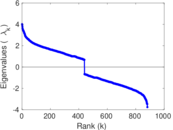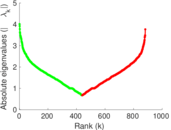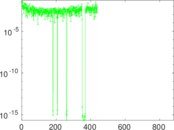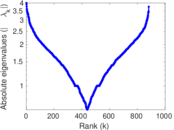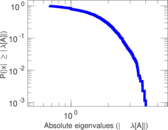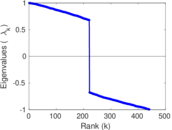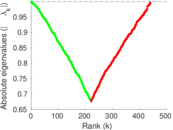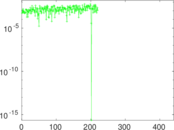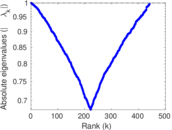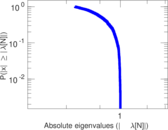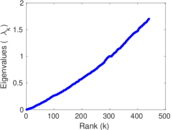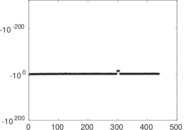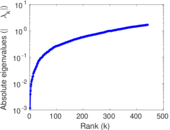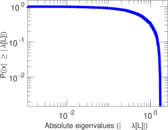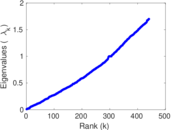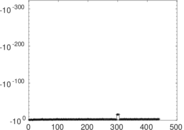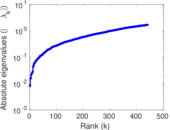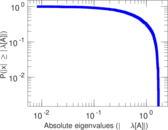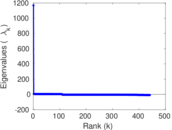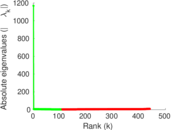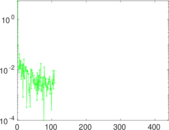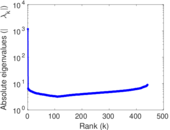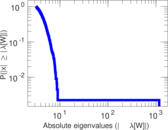This is the international E-road network, a road network located mostly in Europe. The network is undirected; nodes represent cities and an edge between two nodes denotes that they are connected by an E-road.

 Code ET Internal name subelj_euroroad Name Euroroads Data source http://lovro.lpt.fri.uni-lj.si/support.jsp AvailabilityDataset is available for download Consistency checkDataset passed all tests Category Infrastructure network Node meaning City Edge meaning Road Network format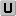Unipartite, undirected Edge typeUnweighted, no multiple edges LoopsDoes not contain loops

Statistics

 Size n = 1,174 Volume m = 1,417 Loop count l = 0 Wedge count s = 2,833 Claw count z = 1,983 Cross count x = 1,308 Triangle count t = 32 Square count q = 41 4-Tour count T4 = 14,494 Maximum degree dmax = 10 Average degree d = 2.413 97 Fill p = 0.002 057 94 Size of LCC N = 1,039 Diameter δ = 62 50-Percentile effective diameter δ0.5 = 16.935 8 90-Percentile effective diameter δ0.9 = 33.340 5 Median distance δM = 17 Mean distance δm = 19.181 2 Gini coefficient G = 0.240 881 Balanced inequality ratio P = 0.412 844 Relative edge distribution entropy Her = 0.984 809 Power law exponent γ = 2.289 90 Tail power law exponent γt = 6.401 00 Tail power law exponent with p γ3 = 6.401 00 p-value p = 0.713 000 Degree assortativity ρ = +0.126 684 Degree assortativity p-value pρ = 1.302 58 × 10−11 Clustering coefficient c = 0.033 886 3 Spectral norm α = 4.010 44 Algebraic connectivity a = 0.001 160 30 Spectral separation |λ1[A] / λ2[A]| = 1.023 07 Non-bipartivity bA = 0.061 239 9 Normalized non-bipartivity bN = 0.004 177 77 Algebraic non-bipartivity χ = 0.008 094 25 Spectral bipartite frustration bK = 0.000 805 549 Controllability C = 103 Relative controllability Cr = 0.087 734 2

Plots

Fruchterman–Reingold graph drawing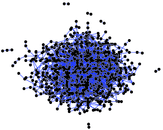Degree distribution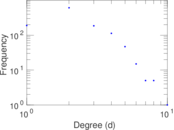Cumulative degree distribution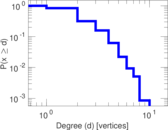Lorenz curve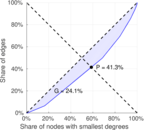Spectral distribution of the adjacency matrix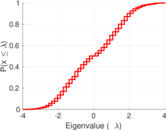Spectral distribution of the normalized adjacency matrix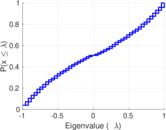Spectral distribution of the Laplacian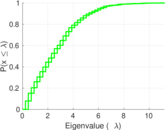Spectral graph drawing based on the adjacency matrix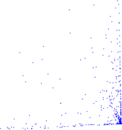Spectral graph drawing based on the Laplacian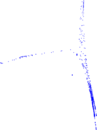Spectral graph drawing based on the normalized adjacency matrixDegree assortativity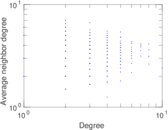Zipf plot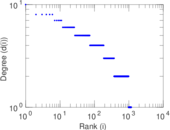Hop distribution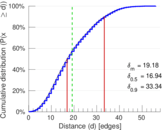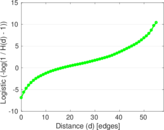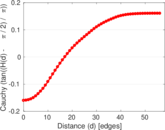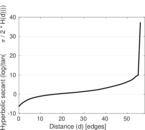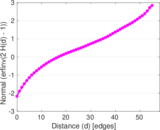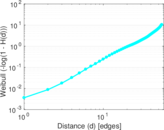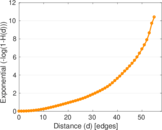Double Laplacian graph drawing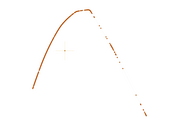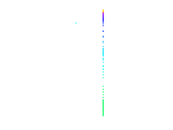Delaunay graph drawing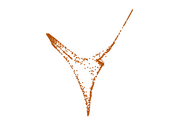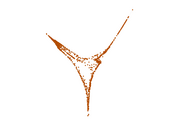Clustering coefficient distribution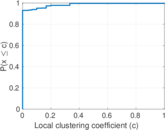Average neighbor degree distribution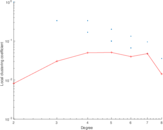SynGraphy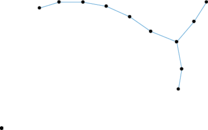Matrix decompositions plots